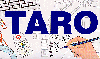The Traffic Accident Reconstruction Origin -Article-[Home] [ARnews] [Contents] [Classified] [Advertisers] [Approach Angles] [E-mail Directory] [Feedback] [Organizations] [Reference Library]

### by Joseph N. Cofone

Sines and Cosines, these common place trigonometric functions appear through the concepts and equations accident reconstructionists use every day. So what do they mean and why do we use them? In the paragraphs that follow an attempt will be made to answer this question.

DISCUSSION

Chances are that any time you are analyzing motion or the application of force you will likely need to employ sines and cosines. Probably the most familiar application where sines and cosines are encountered by the accident reconstructionists is in performing a conservation of linear momentum (COLM) analysis. We will use linear momentum to help us in our understanding of the use of Sines and Cosines in accident reconstruction. But first lets explore some common background terms and concepts.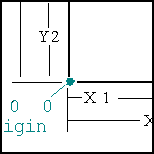Figure 1 We can define any particular point in space with a Cartesian coordinate system. To do this we must first pick an arbitrary point and call it the origin. Next, we must choose an arbitrary direction. This direction is called the positive X direction and defines the X axis. Given these two references the Y axis is drawn perpendicular to the X axis at the origin and we have a two dimensional Cartesian coordinate system. Every point in this Cartesian space can be described with its X and Y coordinate. In Figure 1, point A has the coordinates X1,Y1. Point B has the coordinate X2, Y2.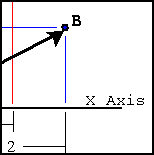Figure 2 If we were to draw a line from the origin to points A and B we can see that each of these lines form different angles with the X axis. We add arrow heads to these lines to remind us that they started at the origin and went to the points A and B. These are "Directed Line Segments". Directed Line segments can also be called Vectors.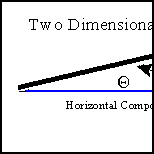Figure 3 Momentum can be graphically represented by a vector. When momentum is represented as a vector, its length (or its magnitude) represents the amount of momentum or Speed x Weight. The angle of the vector represents the direction of the momentum in relation to a fixed coordinate system. Momentum is a vector quantity we use in accident reconstruction. It is a vector quantity because it has a magnitude and direction. Whether the momentum is one dimensional, as in collinear COLM collision (inline) analysis, or two dimensional as in most other cases, we examine the components of the momentum to understand the overall momentum. (See Figure 3).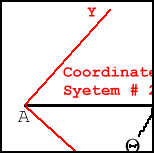Figure 4 Of course one dimensional cases are one dimensional cases by virtue of our choice for the coordinate system. That is, we choose our system coincident with the momentums that exists in our collision. Note the two vectors A in Figure 4 are identical. One has an angle of Theta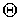. The other has an angle of 0. It is clear that the angle is completely dependent on how we defined our coordinate system. Coordinate system #1 has its X axis parallel to the vector B. Coordinate system #2 has the axis pointed in another direction and consequently B has an angle Theta. Recall that this "fixing of an axis" parallel to an approach angle is the first step performed in any momentum analysis. Though any coordinate system would work, aligning the system paralell with one of the approach vectors greatly simplifies the solution of the momentum problem.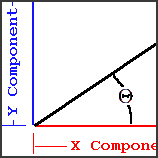Figure 5 Finding the components of a vector in a given coordinate system is the reason we use sines and cosines. In Figure 5 we see that vector D, with coordinates X,Y, has components in both the X and Y direction. Since we drew this vector from the origin to the point X,Y its components are easy to recognize. Vector D's X component is X, and its Y component is Y. Viewing Figure 5 it is important to see that the Y component indicated in light blue is equal in length to the Y Component that is projected onto the Y axis, here labeled in darker blue.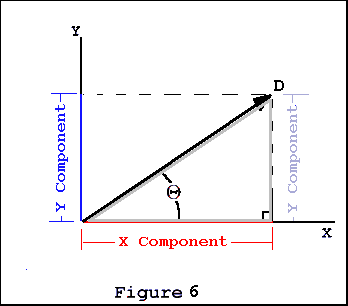Figure 5 is redrawn as Figure 6 emphasising the triangle that is made up of vector D, its X component and its Y component. Several things should be apparent about this triangle.

1. This is a right triangle

2. Since it is a right triangle we also know that the length of D 2 = X2 + Y 2

What might not be as immediately apparent are the trigonometric relationships in this right triangle. Trigonometry tells us that:
1. The X component = D cos()

2. The Y component = D sin()

Notice that we have invoked trigonometry so that we can calculate the length of the components based on the length of the vector and its angle. The idea that the Sine or Cosine of an angle can be used to calculate the unknown side of a triangle (or find a component) answers the question posed by this article: Sines and Cosines- What they are and why we use them.

With these basics ideas behind us we will state the basic Conservation of Linear Momentum principle, then state the relationship in another equivalent way.

The sum of the pre collision momentum is equal to the sum of the post collision momentum.

Modified Definition

The sum of the pre collision momentum components is equal to the sum of the post collision momentum components.

We can simplify this idea further. We will restate the modified definition in a different but still equivalent way.

Equivalent Modified Definition

The sum of the pre collision X components of momentum is equal to the sum of the sum of the post collision X components of momentum. And, the sum of the pre collision Y components of momentum is equal to the sum of the sum of the post collision Y components of momentum .

Using this Equivalent Modified Definition is what we do when we solve a COLM problem. We reduce the momentum vector problem to its components, then solve for them. An example will help illustrate the idea. We will illustrate with a simple momentum collision. For this example we will choose two vehicles that meet at right angles, W1V1 and W2V2. For the sake of simplicity and illustrating this concept, these vehicles will become intertwined and depart as a single unit, (W1+W2)V3.

In Figure 7 we perform the following steps.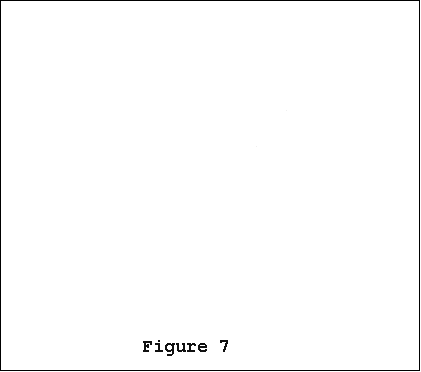• From the scene information we measure the approach and departure angles.

• Based on these measurements and calculations we draw a rough vector diagram. Notice that even though we know the approach angle of both vehicles, and the departure angle, we do not know the magnitude, or length, of these vectors. That is why this is a rough diagram and the vectors are shown as broken lines.

• Using the combined weights of vehicles 1 and 2, and scene information we calculate the post collision momentum of the entangled vehicles, (W1+W2)V3.

• We align our vector diagram on a coordinate axis of our choosing.

In Figure 8 we perform the following steps.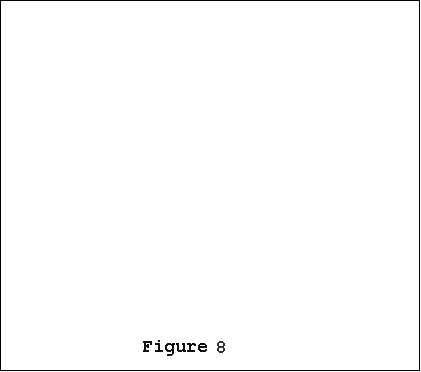• We use (W1+W2)V3sin() to calculate the Y component of post collision momentum

• We equate the post collision Y component of momentum to the pre collision Y component of momentum. They are equal.

In Figure 9 we perform the same steps as we did in figure 8 except we look at X components.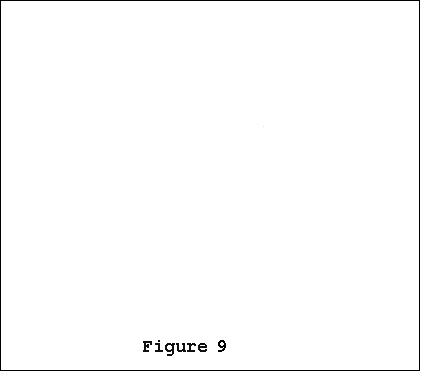• We calculate the X component of post collision momentum as (M1+M2)V3cos()

• We now know the X component of pre collision momentum. It is equal to the post collision X component calculated immediately above.

It is worth noting that this example problem was chosen to make it easy to visualize equating momentums before and after the collision. Though other problems with separate departures and acute or obtuse approaches would be more complicated, all the relationships stated above still hold. In these cases care must be taken to remember the sense (direction) of each momentum component. Adding or subtracting as indicated will result in correct answers that can be checked graphically.

CLOSING

This article has shown that Sines and Cosines enable us to examine the individual components of momentum. They allow us to solve for post impact momentum components. Then applying the COLM principle, we can equate this to the pre collision momentum components.

Beyond momentum Sines and Cosines are useful in finding components of any vector quantity. Acceleration, Velocity and Force all lend themselves to this type of component analysis. Trigonometry offers some powerful tools for the reconstructionist. Sines and Cosines are just the most visible ones.

[Home] [ARnews] [Contents] [Classified] [Advertisers] [Approach Angles] [E-mail Directory] [Feedback] [Organizations] [Reference Library]